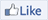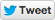18 June 2013Article A giant step for twin primesThree and five. Five and seven. Eleven and thirteen. Prime numbers often appear as twins, only two apart. For hundreds of years, mathematicians have wondered – is there a biggest pair of twin primes, or does the list of twins keep going forever?

A lot of small primes are also twin primes. But as you look at bigger and bigger primes, the average gap between them also gets bigger. Eventually, prime numbers are one-in-a-million, one-in-a-billion, or even less common.

However there’s also evidence for endless twin primes. Although they can be hard to find, mathematicians have found some truly large prime twins. The largest pair of twin primes currently known has over 200 000 digits in each number. These numbers are so large that mathematicians write them as a calculation, rather than a number.

We don’t know how many twin primes exist. But recently, Chinese mathematician Yitang Zhang made a very big step towards an answer. His work looks at more distant pairings. In Yitang’s work, two primes are paired if they differ by less than 70 million – so twin primes are included, but so are primes that differ by 3 347 642, for example. Yitang’s proof shows there is an infinite number of these more distant pairings.

You might think that such distant pairs are a long way from twins differing by two. But number theorists are excited by this discovery. Yitang’s result strongly suggests that the list of twin primes goes on forever. Several mathematicians are already improving the proof to make the pairs closer together. Things are looking good for an endless list of twins.

What is a prime number?
A simple explanation of the twin prime conjecture
Prime number breakthrough by unknown professor
Several prominent mathematicians improve the proof in this conversation (very advanced)Try this Napier's bonesArrange strips to spell out your number on the first line.The units digit of the answer is the last digit on the row.Add together the next two digits on the bones to get the second-last digit of your answer.These boxes add to 10. Write down the 0, and a note to carry the 1.Remember to carry 1 when the previous digits added up to 10 or more.### What to do

1. Thoroughly stick each copy of the Napier’s bones template to some cardboard. This isn’t necessary, but it makes the bones a lot easier to use.
2. The template consists of 10 strips (or bones) separated by heavy black lines. Cut along all the heavy black lines to separate your bones.

### Multiplying using Napier’s bones

1. Think of a four digit number – something like 4670.
2. Look at the top numbers on your bones. Grab a bone with the first digit of your four digit number, then one with the second, then one with the third and one with the fourth. Put them side by side in order, on the board if you wish.
3. Think of a one digit number to multiply your four digit number by. Then count down the rows to get to that number – so if you picked 3, read the third row.
4. The row will look something like 1/2 1/8 2/1 0/0. Start from the right side. Write down the right-most digit on a separate piece of paper – in this case it is 0.
5. Then read the next two digits to the left – they are located diagonally on different bones. Add them together and write the result down to the left of your last digit. In this case, the two digits add to get 1. This means our example will read ‘10’.
6. Continue to read pairs of digits, adding them together and writing them to the left of the previous digits. If you get an answer with two digits, only write down the units on your paper. When you add the next two digits together, add a 1 to the answer before writing it down. As an example, for the third digit of 4670 x 3 we would add 8 + 2 = 10. Write down 0, and remember to carry a 1.
7. When you reach the left side of the column, there will be a single digit by itself. Write this down, remembering to add 1 if you need to carry. You’ve finished the multiplication!
8. If you have a calculator handy, you can check your answer. In our example, the answer is 14 010.

### What’s happening?

Napier’s bones were invented about 400 years ago by John Napier. They were popular for many years, until much faster mechanical calculators started to appear around 1900.

They are remarkably simple – each bone is simply a times table for the digit at the top. Reading down the six rod, you see 6, 12, 18, 24, 30, etc. To simplify carrying, the diagonal lines separate the tens and ones digits.

### Applications

Napier’s bones are great for multiplication, but you can also use them to do division. Napier’s bones can also be used to do larger multiplications, and even to find square roots, although you need a separate square root bone to make it possible.

Napier’s bones, with an interactive at the bottom of the pageBrainteaser questionTwin primes differ by 2. So 3 and 5 are twin primes, and so are 17 and 19.   How many primes differ by 1?

Did you know? Many species of cicada pupate – that is they stay underground to mature – for a prime number of years. The green grocer and yellow Monday cicadas from south-eastern Australia pupate for seven years and in America there are species that pupate for 13 and 17 years!WebsitesThere are hundreds of thousands of sequences that mathematicians have discovered. Two mathematicians decided to review some.

### See maths!

There’s maths all around, if you know where to look. This image gallery has heaps of photos of mathematical things.

 Brainteaser answerOnly one pair of primes differs by 1, that’s 2 and 3.   If a pair of numbers differs by 1, then one of them is even and the other is odd. The number 2 is the only even prime, so we only have to check for primes adjacent to 2.   The numbers 1 and 2 also differ by 1, but mathematicians don’t consider 1 to be a prime.

Our partners The mission of the Australian Bureau of Statistics is to assist and encourage informed decision making, research and discussion within governments and the community, by leading a high quality, objective and responsive national statistical service. The Australian Mathematical Sciences Institute's mission is the radical improvement of mathematical sciences capacity and capability in the Australian community through the support of high quality mathematics education for all young Australians.